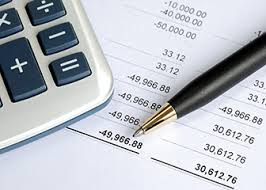Introduction

Double-declining method is used to record higher depreciation expense in the earlier stages of an asset’s life and respectively lower depreciation expense as the asset ages. This method is sometimes called accelerated depreciation method. The double declining balance calculation does not consider the salvage value in the depreciation of each period however, if the book value will fall below the salvage value, the last period might be adjusted so that it ends at the salvage value. When double declining balance method does not fully depreciate an asset by the end of its life, variable declining balance method might be used instead.

## Double-declining methodDouble-declining method applies a constant rate (double of the straight-line rate) to the net book value of the asset and produces a decreasing annual depreciation expense over the asset useful life. The decrease in depreciation relates to the decrease in the asset’s net book value in each subsequent period.

To determine depreciation expense under double-declining method, accountants do the following:

1. Calculate the straight-line rate. It is calculated by dividing 100% by the number of years the asset is expected to be in use. So, in Mr. Serfy’s example, the rate is 25% (100% / 4 years).
2. Determine the double-declining balance rate by multiplying the straight-line rate by two: 25% x 2 = 50%.
3. Apply the double-declining balance rate to the book (carrying) value of the asset at the beginning of each period.

The table below depicts the depreciation expense computations under double-declining balance method:

##### Illustration : Schedule of double-declining depreciation for Mr. Serfy’s computer
 Year Book Value at Beginning of Period x Double the Straight- Line Rate = Annual Depreciation Expense 20X7 (23,000 – 0) x 50% = \$11,500 20X8 (23,000 – 11,500) x 50% = \$5,750 20X9 (23,000 – 17,250) x 50% = \$2,875 2,750 20X0 (23,000 – 20,000) x 50% = \$1,500 0

Note that the book value of an asset cannot be depreciated below its salvage value. The computer historical cost is \$23,000 and the salvage value is \$3,000, so the amount to be depreciated is \$20,000. Because \$17,250 ( \$11,500 + \$5,750) is depreciated after two periods 20X7 and 20X8, only \$2,750 more (\$20,000 – \$17,250) can be depreciated. Accordingly, the \$2,875 is ignored in the third year because it exceeds \$2,750 limit. Basing on the aforementioned considerations, the \$1,500 is also ignored in the fourth year. Observe that the depreciation expense amounts diminished as the computer aged.